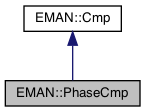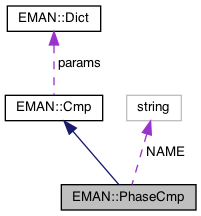EMAN2
EMAN::PhaseCmp Class Reference

Amplitude weighted mean phase difference (radians) with optional SNR weight. More...

`#include <cmp.h>`

Inheritance diagram for EMAN::PhaseCmp:[legend]
Collaboration diagram for EMAN::PhaseCmp:[legend]

## Public Member Functions

float cmp (EMData *image, EMData *with) const
To compare 'image' with another image passed in through its parameters. More...

string get_name () const
Get the Cmp's name. More...

string get_desc () const

TypeDict get_param_types () const
Get Cmp parameter information in a dictionary. More...Public Member Functions inherited from EMAN::Cmp
virtual ~Cmp ()

virtual Dict get_params () const
Get the Cmp parameters in a key/value dictionary. More...

virtual void set_params (const Dict &new_params)
Set the Cmp parameters using a key/value dictionary. More...

static CmpNEW ()

## Static Public Attributes

static const string NAME = "phase"Protected Member Functions inherited from EMAN::Cmp
void validate_input_args (const EMData *image, const EMData *with) constProtected Attributes inherited from EMAN::Cmp
Dict params

## Detailed Description

Amplitude weighted mean phase difference (radians) with optional SNR weight.

SNR should be an array as returned by ctfcurve() 'with' should be the less noisy image, since it's amplitudes will be used to weight the phase residual. 2D only.

Use Phase Residual as a measure of similarity Differential phase residual (DPR) is a measure of statistical dependency between two averages, computed over rings in Fourier space as a function of ring radius (= spatial frequency, or resolution)

Definition at line 636 of file cmp.h.

## ◆ cmp()

 float PhaseCmp::cmp ( EMData * image, EMData * with ) const
virtual

To compare 'image' with another image passed in through its parameters.

An optional transformation may be used to transform the 2 images.

Parameters
 image The first image to be compared. with The second image to be comppared.
Returns
The comparison result. Smaller better by default

Implements EMAN::Cmp.

Definition at line 1222 of file cmp.cpp.

1223{
1224 ENTERFUNC;
1225
1226 int snrweight = params.set_default("snrweight", 0);
1227 int snrfn = params.set_default("snrfn",0);
1228 int ampweight = params.set_default("ampweight",0);
1230 float minres = params.set_default("minres",500.0f);
1231 float maxres = params.set_default("maxres",10.0f);
1232
1233 if (snrweight && snrfn) throw InvalidCallException("SNR weight and SNRfn cannot both be set in the phase comparator");
1234
1235 EMData *image_fft = NULL;
1236 EMData *with_fft = NULL;
1237
1238 int ny = image->get_ysize();
1239// int np = (int) ceil(Ctf::CTFOS * sqrt(2.0f) * ny / 2) + 2;
1240 int np = 0;
1241 vector<float> snr;
1242
1243 // weighting based on SNR estimate from CTF
1244 if (snrweight) {
1245 Ctf *ctf = NULL;
1246 if (!image->has_attr("ctf")) {
1247 if (!with->has_attr("ctf")) throw InvalidCallException("SNR weight with no CTF parameters");
1248 ctf=with->get_attr("ctf");
1249 }
1250 else ctf=image->get_attr("ctf");
1251
1252 float ds=1.0f/(ctf->apix*ny);
1253 snr=ctf->compute_1d(ny,ds,Ctf::CTF_SNR); // note that this returns ny/2 values
1254 for (int i=0; i<snr.size(); i++) {
1255 if (snr[i]<=0) snr[i]=0.001; // make sure that points don't get completely excluded due to SNR estimation issues, or worse, contribute with a negative weight
1256 }
1257 if(ctf) {delete ctf; ctf=0;}
1258 np=snr.size();
1259 }
1260 // weighting based on empirical SNR function (not really good)
1261 else if (snrfn==1) {
1262 np=ny/2;
1263 snr.resize(np);
1264
1265 for (int i = 0; i < np; i++) {
1266 float x2 = 10.0f*i/np;
1267 snr[i] = x2 * exp(-x2);
1268 }
1269 }
1270 // no weighting
1271 else {
1272 np=ny/2;
1273 snr.resize(np);
1274
1275 for (int i = 0; i < np; i++) snr[i]=1.0;
1276 }
1277
1278 // Min/max modifications to weighting
1279// float pmin,pmax;
1280
1281 float pmin = params.set_default("pmin",0.0f);
1282 float pmax = params.set_default("pmax",0.0f);
1283 if (minres>0 && pmin==0) pmin=((float)image->get_attr("apix_x")*image->get_ysize())/minres; //cutoff in pixels, assume square
1284// else pmin=0;
1285 if (maxres>0 && pmax==0) pmax=((float)image->get_attr("apix_x")*image->get_ysize())/maxres;
1286// else pmax=0;
1287
1288// printf("%f\t%f\n",pmin,pmax);
1289
1290 // We use 'soft' edges for the Fourier cutoffs to minimize issues with pixel interpolation
1291 for (int i = 0; i < np; i++) {
1292 if (pmin>0) snr[i]*=(tanh(5.0f*(i-pmin)/pmin)+1.0f)/2.0f;
1293 if (pmax>0) snr[i]*=(1.0f-tanh(i-pmax))/2.0f;
1294// printf("%d\t%f\n",i,snr[i]);
1295 }
1296
1298 image_fft=image->copy();
1299 with_fft=with->copy();
1300
1301 if (image_fft->is_complex()) image_fft->do_ift_inplace();
1302 if (with_fft->is_complex()) with_fft->do_ift_inplace();
1303
1304 int sz=image_fft->get_xsize()*image_fft->get_ysize()*image_fft->get_zsize();
1305 float *d1=image_fft->get_data();
1306 float *d2=with_fft->get_data();
1307
1308 for (int i=0; i<sz; i++) {
1309 if (d1[i]==0.0 || d2[i]==0.0) { d1[i]=0.0; d2[i]=0.0; }
1310 }
1311
1312 image_fft->update();
1313 with_fft->update();
1314 image_fft->do_fft_inplace();
1315 with_fft->do_fft_inplace();
1316 image_fft->set_attr("free_me",1);
1317 with_fft->set_attr("free_me",1);
1318 }
1319 else {
1320 if (image->is_complex()) image_fft=image;
1321 else {
1322 image_fft=image->do_fft();
1323 image_fft->set_attr("free_me",1);
1324 }
1325
1326 if (with->is_complex()) with_fft=with;
1327 else {
1328 with_fft=with->do_fft();
1329 with_fft->set_attr("free_me",1);
1330 }
1331 }
1332// image_fft->ri2ap();
1333// with_fft->ri2ap();
1334
1335 const float *const image_fft_data = image_fft->get_const_data();
1336 const float *const with_fft_data = with_fft->get_const_data();
1337 double sum = 0;
1338 double norm = FLT_MIN;
1339 size_t i = 0;
1340// int nx=image_fft->get_xsize();
1341 ny=image_fft->get_ysize();
1342 int nz=image_fft->get_zsize();
1343 int nx2=image_fft->get_xsize()/2;
1344 int ny2=image_fft->get_ysize()/2;
1345// int nz2=image_fft->get_zsize()/2;
1346
1347 // This can never happen any more...
1348 if (np==0) {
1349 for (int z = 0; z < nz; z++){
1350 for (int y = 0; y < ny; y++) {
1351 for (int x = 0; x < nx2; x ++) {
1352 float a;
1353 if (ampweight) a= (float)hypot(with_fft_data[i],with_fft_data[i+1]);
1354 else a=1.0f;
1355 sum += Util::angle_err_ri(image_fft_data[i],image_fft_data[i+1],with_fft_data[i],with_fft_data[i+1]) * a;
1356 norm += a;
1357 i += 2;
1358 }
1359 }
1360 }
1361
1362 }
1363 else if (nz==1) {
1364 for (int y = 0; y < ny; y++) {
1365 for (int x = 0; x < nx2; x ++) {
1366 int r=Util::hypot_fast_int(x,y>ny/2?ny-y:y);
1367 if (r>=ny2) { i+=2; continue; } // we only have snr values to the box radius
1368
1369 float a;
1370 if (ampweight) a= (float)hypot(with_fft_data[i],with_fft_data[i+1]);
1371 else a=1.0f;
1372 a*=snr[r];
1373 sum += Util::angle_err_ri(image_fft_data[i],image_fft_data[i+1],with_fft_data[i],with_fft_data[i+1]) * a;
1374 norm += a;
1375 i += 2;
1376 }
1377 }
1378 }
1379 else {
1380 for (int z = 0; z < nz; z++){
1381 for (int y = 0; y < ny; y++) {
1382 for (int x = 0; x < nx2; x ++) {
1383 int r=(int)Util::hypot3(x,y>ny/2?ny-y:y,z>nz/2?nz-z:z);
1384 if (r>=ny2) { i+=2; continue; } // we only have snr values to the box radius
1385
1386 float a;
1387 if (ampweight) a= (float)hypot(with_fft_data[i],with_fft_data[i+1]);
1388 else a=1.0f;
1389 a*=snr[r];
1390 sum += Util::angle_err_ri(image_fft_data[i],image_fft_data[i+1],with_fft_data[i],with_fft_data[i+1]) * a;
1391 norm += a;
1392 i += 2;
1393 }
1394 }
1395 }
1396
1397 }
1398
1399 EXITFUNC;
1400
1401 if( image_fft->has_attr("free_me") )
1402 {
1403 delete image_fft;
1404 image_fft = 0;
1405 }
1406 if( with_fft->has_attr("free_me") )
1407 {
1408 delete with_fft;
1409 with_fft = 0;
1410 }
1411#if 0
1412 return (1.0f - sum / norm);
1413#endif
1414 return (float)(sum / norm);
1415}
Dict params
Definition: cmp.h:132
Ctf is the base class for all CTF model.
Definition: ctf.h:60
float apix
Definition: ctf.h:88
virtual vector< float > compute_1d(int size, float ds, CtfType t, XYData *struct_factor=0)=0
@ CTF_SNR
Definition: ctf.h:68
type set_default(const string &key, type val)
Default setting behavior This can be achieved using a template - d.woolford Jan 2008 (before there wa...
Definition: emobject.h:569
EMData stores an image's data and defines core image processing routines.
Definition: emdata.h:82
static short hypot_fast_int(int x, int y)
Euclidean distance in 2D for integers computed fast using a cached lookup table.
Definition: util.cpp:764
static float angle_err_ri(float r1, float i1, float r2, float i2)
Calculate the angular phase difference between two r/i vectors.
Definition: util.h:1099
static float hypot3(int x, int y, int z)
Euclidean distance function in 3D: f(x,y,z) = sqrt(x*x + y*y + z*z);.
Definition: util.h:827
#define InvalidCallException(desc)
Definition: exception.h:348
#define ENTERFUNC
Definition: log.h:48
#define EXITFUNC
Definition: log.h:49
#define y(i, j)
Definition: projector.cpp:1516
#define x(i)
Definition: projector.cpp:1517

## ◆ get_desc()

 string EMAN::PhaseCmp::get_desc ( ) const
inlinevirtual

Implements EMAN::Cmp.

Definition at line 646 of file cmp.h.

647 {
648 return "Mean phase difference";
649 }

## ◆ get_name()

 string EMAN::PhaseCmp::get_name ( ) const
inlinevirtual

Get the Cmp's name.

Each Cmp is identified by a unique name.

Returns
The Cmp's name.

Implements EMAN::Cmp.

Definition at line 641 of file cmp.h.

642 {
643 return NAME;
644 }
static const string NAME
Definition: cmp.h:668

References NAME.

## ◆ get_param_types()

 TypeDict EMAN::PhaseCmp::get_param_types ( ) const
inlinevirtual

Get Cmp parameter information in a dictionary.

Each parameter has one record in the dictionary. Each record contains its name, data-type, and description.

Returns
A dictionary containing the parameter info.

Implements EMAN::Cmp.

Definition at line 656 of file cmp.h.

657 {
658 TypeDict d;
659 d.put("snrweight", EMObject::INT, "If set, the SNR of 'this' will be used to weight the result. If 'this' lacks CTF info, it will check 'with'. (default=0)");
660 d.put("snrfn", EMObject::INT, "If nonzero, an empirical function will be used as a radial weight rather than the true SNR. (1 - exp decay)'. (default=0)");
661 d.put("ampweight", EMObject::INT, "If set, the amplitude of 'with' will be used as a weight in the averaging'. (default=0)");
662 d.put("zeromask", EMObject::INT, "Treat regions in either image that are zero as a mask");
663 d.put("minres", EMObject::FLOAT, "Lowest resolution to use in comparison (soft cutoff). Requires accurate A/pix in image. <0 disables. Default=500");
664 d.put("maxres", EMObject::FLOAT, "Highest resolution to use in comparison (soft cutoff). Requires accurate A/pix in image. <0 disables. Default=10");
665 return d;
666 }

References EMAN::EMObject::FLOAT, EMAN::EMObject::INT, and EMAN::TypeDict::put().

## ◆ NEW()

 static Cmp * EMAN::PhaseCmp::NEW ( )
inlinestatic

Definition at line 651 of file cmp.h.

652 {
653 return new PhaseCmp();
654 }

## ◆ NAME

 const string PhaseCmp::NAME = "phase"
static

Definition at line 668 of file cmp.h.

Referenced by get_name().

The documentation for this class was generated from the following files: